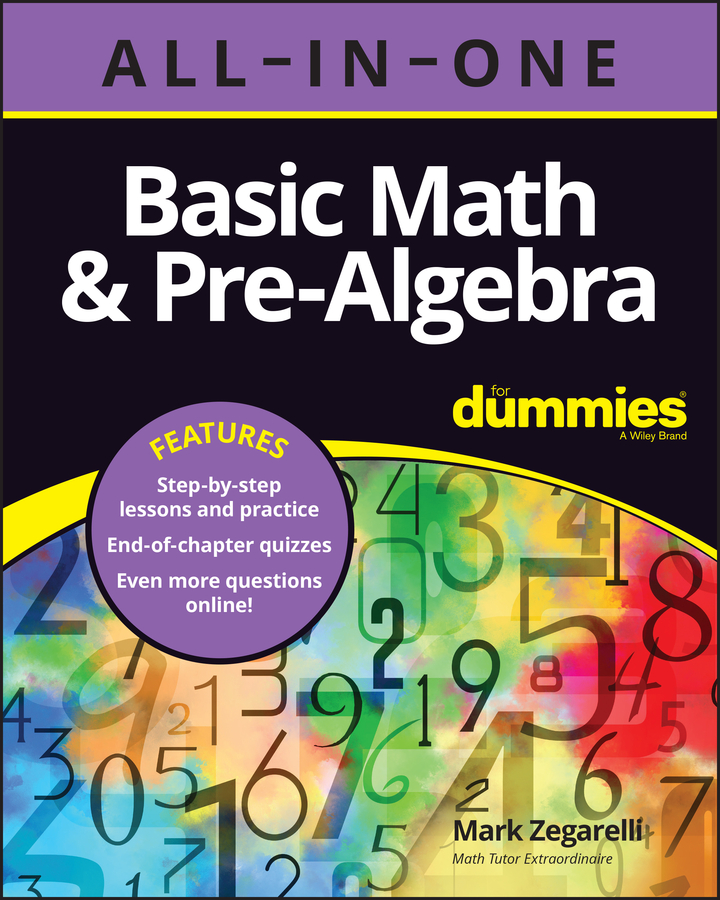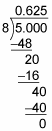##### Basic Math & Pre-Algebra All-in-One For Dummies (+ Chapter Quizzes Online)Converting a fraction to a percent is a simple two-step process. The first example below shows how to convert a simple fraction, and the second example shows how to convert a more complex fraction using long division.

This first example shows you the basic steps:

1. Convert the fraction to a decimal.

For example, suppose you want to convert the fraction 4/5 to a percent. To convert 4/5 to a decimal, you can divide the numerator by the denominator:

4/5 = 0.8

2. Convert this decimal to a percent.

Convert 0.8 to a percent by moving the decimal point two places to the right and adding a percent sign.

0.8 = 80%

This second example shows how to convert a more complex fraction, 5/8, to a percent using long division:

1. Convert 5/8 to a decimal by dividing the numerator by the denominator:Therefore, 5/8 = 0.625.

2. Convert 0.625 to a percent by moving the decimal point two places to the right and adding a percent sign (%):

0.625 = 62.5%